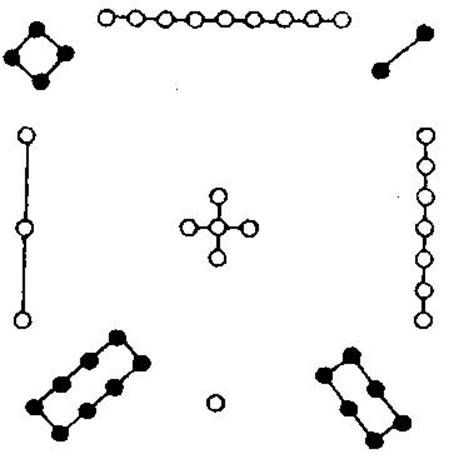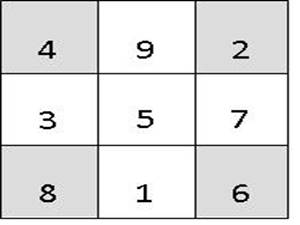解读洛书九宫图数学奥秘

大禹治水时，神龟出于洛水，龟背图案是从一到九个数，分别排列在九个位置，也称洛书，有口诀：戴九履一，左三右七，二四为肩，六八为足，五居中央，正龟背之象也。五作为九个自然数序列的中间，居洛书中间位置，偶数二、四、六、八为阴，为地之道，用黑点标识，处于四隅方位（也称四维，东北、东南、西南、西北四个方位），奇数一、三、七、九为阳为天之道，用白点标识，处于四正方位（正东、正西、正南、正北）。洛书图案是采用黑白点的数量来排列，在此基础上，后来又演化出易经相关分支中常用的九宫八门图，简称九宫图。九宫图排列分布遵循严格数学算法和数字序列规律，看起来简单九个数字，其中的隐藏秩序却变化无穷，除了在算术方面千变万化皆天机外，九宫图还被用于天体九星运行规律的研究。九宫图在企业管理思维方式以及环境的优化布局方面，对于开阔我们的思路与制定企业未来发展战略具有重要意义。洛书九宫图的特点是从19自然数排列在九宫格子中，在算术方面，所有的横行、竖行和两个对角线数字之和均等于15，数字在九宫格内的排列必须按照上面提及的口诀，除此以外没有其他答案。奇数和偶数交错排列，四隅为偶数，中间和四正为奇数。
九宫图的数字变幻无穷，其中四组等差数列：   ，均以5为中心逆时针旋转，各组数列的公差分别为1234，形成自然序列。如果将四隅的偶数整体顺时针旋转进入四正位置，奇数与偶数之差等于5。这些规律一般中小学生都可以发现，并不足为奇。九宫图更为神奇的是：除了横行、竖行和对角线各数字之和等于15以外，无论哪一行列或者对角线的数字做正向和逆向循环组合形成三组数字相加都相等。我们以第一行的、第二行的和第三行的加以举例说明。

1）当把这三个数两两循环组合，形成三个两位数相加时，数值和依然相等。具体操作如下：

49+92+24=35+57+73=81+16+68=165

29+94+42=75+53+37=61+18+86=165

2）当把这三个数三三循环组合，形成三个三位数相加时，数值和依然相等。具体操作如下：

492+924+249=357+573+735=816+168+681=1665

294+942+429=753+537+375=618+186+861=1665

3）当把三个数四四循环组合，形成三个四位数相加时，数值和依然相等。具体操作如下：

4924+9249+2492=3573+5735+7357=8168+1681+6816=16665

2942+9429+4294=7537+5375+3753=6186+1861+8618=16665

(1×2×3+4×5×6+7×8×9)-(1×4×7+2×5×8+3×6×9)=360

九宫图数字组合排列的规律不得不让人发自内心的惊叹：中国古圣先贤的智慧真是如此的奥妙无穷、深不可测，做一名中国人，我骄傲，我自豪！从以上论述的九宫图变化规律看，数字5永远是九宫图的中位原点，或者称之为全息元点，它始终显性或者隐性的存在于不同的数字序列里面，在各种数字按照一定规律组合排列的变换过程中，始终与它息息相关，从没有分离过。由此可知，任何事物都一定有类似九宫图数字5的中位，并以中位为核心由一开始变化，从而造就了多彩多姿的大千世界，但无论经历怎样的变化，总可以由中位数5控制回归到起点1，此即为一多互摄，万法归一。所以，孔子说：参乎，悟道一以贯之。老子说：昔之得一者：天得一以清；地得一以宁；神得一以灵；谷得一以盈；侯得一以为天下正。